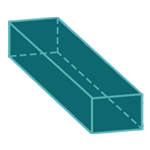Report a Problem
Suggestions

#Calculate The Volume of A Cuboid

Last updated: Saturday, April 29, 2023

A cuboid is a three-dimensional shape that has six rectangular faces and 90-degree angles between them. It is a prism with a rectangular base and has three pairs of congruent rectangular faces.

Cuboids are commonly found in everyday objects such as books, cereal boxes, and bricks. They are also frequently used in architecture, where they can be used to design rectangular buildings or create rectangular windows and doors. In engineering, cuboids are used to design boxes for shipping and storing goods. In the medical field, cuboids are used to model the shape of organs such as the liver, pancreas, and kidney.

Easily calculate the volume of a cuboid with step-by-step guidance using our free calculator below.

The formula for determining the volume of a cuboid is defined as:
$$V$$ $$=$$ $$L$$ $$\cdot$$ $$W$$ $$\cdot$$ $$D$$
$$V$$: the volume of the rectangular prism/cuboid
$$L$$: the length of the longer side
$$W$$: the length of the shorter side
$$D$$: the length of the depth
The SI unit of volume is: $$cubic \text{ } meter\text{ }(m^3)$$

## Find $$V$$

Use this calculator to determine the volume of a rectangular prism/cuboid when the length of all sides are given.
Hold & Drag
CLOSE
the length of the longer side
$$L$$
$$meter$$
the length of the shorter side
$$W$$
$$meter$$
the length of the depth
$$D$$
$$meter$$
Bookmark this page or risk going on a digital treasure hunt again